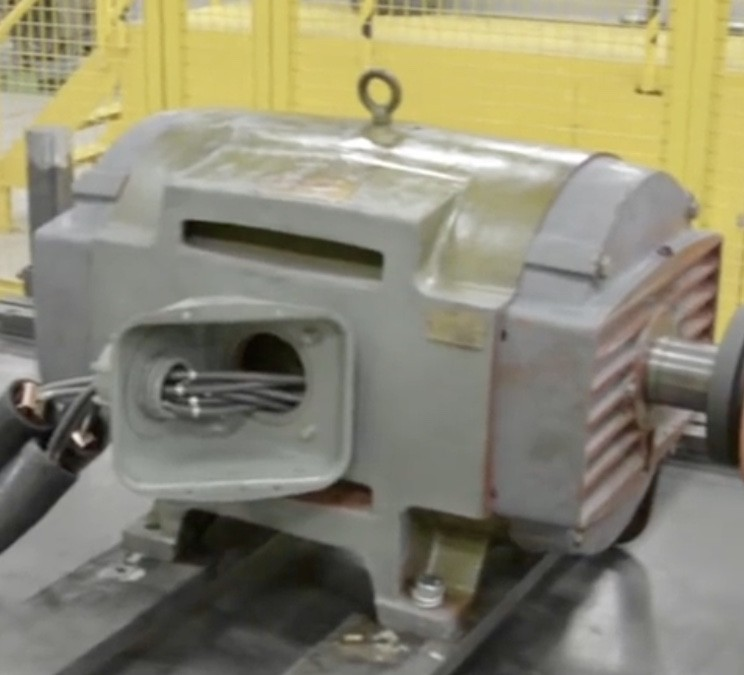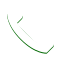# How to Determine a Motor’s HorsepowerWhen sizing a motor for any application there are a lot of factors to consider. Requirements such as speed, torque, frame-size, ramp-up and load all need to be carefully considered. But the first consideration when choosing a motor is understandably how much work can be performed by said motor. The amount of “work” an electric motor can perform is measured in horsepower. When assisting a customer with sizing a motor we often get asked how to determine horsepower because some motor data plates do not clearly state this value. Luckily by using a simple bit of math you can quickly determine horsepower using minimal information. Specifically, the amperage and voltage rating of a motor.

Step One: Determine your Motor’s Wattage

The first step toward determining horsepower is first determining another value by which rate of work is measure called a watt. Named after the famous Scottish inventor James Watt, the watt is a unit of measure that is used to quantify energy transfer in a system. To determine wattage in a motor you must multiply amperage rating by the voltage rating.

V X A = W

Example: 460V X 30A = 13,800 Watts

Step Two: Factor in Efficiency Rating

At its core an electric motor’s job is converting electrical energy into mechanical energy a machine can use to perform work. Unfortunately, no motor is 100% efficient and there are inherent losses to work potential that must be factored in. When listed on a motor data plate this value is most often represented as a percentage. When you see a motor efficiency rating you must convert from a percentage to a decimal for the purposes of this equation. For instance 85% efficiency would be .85 efficiency. Add it to your wattage calculation like so:

V X A X E = W

Example: 460V X 30A X .85 = 11,730

Step Three: Converting Wattage into Horse Power

Lastly, we need to convert wattage into horsepower. 756 watts roughly equal one horsepower. Taking the example above we can take our calculated wattage of 11,730 and divide it by 756. What we end up with is 15.515 or right around 15 Horsepower rounded down. That would mean if we had an example motor rated at 460 volts, and 30 Amps with an efficiency rating of 85 percent this motor would be a 15 horsepower motor.

If you need help with your motor, whether with sizing or if your in need of having it repaired, the professionals at Global Electronic Services are here to help! Be sure to visit us online at gesrepair.com or call us at 1-877-249-1701 to learn more about our services. We’re proud to offer Surplus, Complete Repair and Maintenance on all types of Industrial Electronics, Servo Motors, AC and DC Motors, Hydraulics and Pneumatics. Please subscribe to our YouTube page and Like Us on Facebook! Thank you!Call for Help